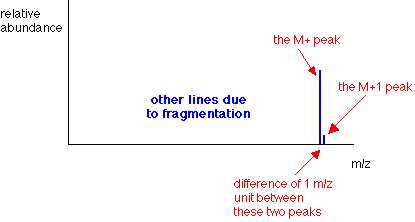Need help? Email help@shout.education

# Mass Spectra – The M+1 Peak

This page explains how the M+1 peak in a mass spectrum can be used to estimate the number of carbon atoms in an organic compound.

Note: This is a small corner of mass spectrometry. It would be a good idea not to attack this page unless you have a reasonable idea about how a mass spectrum is produced and the sort of information you can get from it. If you haven't already done so, explore the mass spectrometry menu before you go on.

## What Causes the M+1 Peak?

### What is an M+1 Peak?

If you had a complete (rather than a simplified) mass spectrum, you will find a small line 1 m/z unit to the right of the main molecular ion peak. This small peak is called the M+1 peak.In questions at this level (UK A-level or its equivalent), the M+1 peak is often left out to avoid confusion – particularly if you were being asked to find the relative formula mass of the compound from the molecular ion peak.

### The Carbon-13 Isotope

The M+1 peak is caused by the presence of the 13C isotope in the molecule. 13C is a stable isotope of carbon – don't confuse it with the 14C isotope which is radioactive. Carbon-13 makes up 1.11% of all carbon atoms.

If you had a simple compound like methane, CH4, approximately 1 in every 100 of these molecules will contain carbon-13 rather than the more common carbon-12. That means that 1 in every 100 of the molecules will have a mass of 17 (13 + 4) rather than 16 (12 + 4).

The mass spectrum will therefore have a line corresponding to the molecular ion [13CH4]+ as well as [12CH4]+.

The line at m/z = 17 will be much smaller than the line at m/z = 16 because the carbon-13 isotope is much less common. Statistically you will have a ratio of approximately 1 of the heavier ions to every 99 of the lighter ones. That's why the M+1 peak is much smaller than the M+ peak.

## Using the M+1 Peak

### What Happens When There is More Than 1 Carbon Atom in the Compound?

Imagine a compound containing 2 carbon atoms. Either of them has an approximately 1 in 100 chance of being 13C.There's therefore a 2 in 100 chance of the molecule as a whole containing one 13C atom rather than a 12C atom – which leaves a 98 in 100 chance of both atoms being 12C.

That means that the ratio of the height of the M+1 peak to the M+ peak will be approximately 2 : 98. That's pretty close to having an M+1 peak approximately 2% of the height of the M+ peak.

Note: You might wonder why both atoms can't be carbon-13, giving you an M+2 peak. They can – and do! But statistically the chance of both carbons being 13C is approximately 1 in 10,000. The M+2 peak will be so small that you couldn't observe it.

### Using the Relative Peak Heights to Predict the Number of Carbon Atoms

#### If there are small numbers of carbon atoms

If you measure the peak height of the M+1 peak as a percentage of the peak height of the M+ peak, that gives you the number of carbon atoms in the compound.

We've just seen that a compound with 2 carbons will have an M+1 peak approximately 2% of the height of the M+ peak.

Similarly, you could show that a compound with 3 carbons will have the M+1 peak at about 3% of the height of the M+ peak.

#### With larger numbers of carbon atoms

The approximations we are making won't hold with more than 2 or 3 carbons. The proportion of carbon atoms which are 13C isn't 1% – it's 1.11%. And the appoximation that a ratio of 2 : 98 is about 2% doesn't hold as the small number increases.

Consider a molecule with 5 carbons in it. You could work out that 5.55 (5 x 1.11) molecules will contain 1 13C to every 94.45 (100 – 5.55) which contain only 12C. If you convert that to how tall the M+1 peak is as a percentage of the M+ peak, you get an answer of 5.9% (5.55/94.45 x 100). That's close enough to 6% that you might assume wrongly that there are 6 carbon atoms.

Above 3 carbon atoms, then, you shouldn't really be making the approximation that the height of the M+1 peak as a percentage of the height of the M+ peak tells you the number of carbons – you will need to do some fiddly sums!